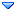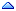GSI Forum
GSI Helmholtzzentrum für Schwerionenforschung

[SOLVED] PAnyDistribution Add Multiple equation Sat, 29 September 2012 07:22Michael Kunkel Messages: 53Registered: June 2011 continuous participant From: *hr.hr.cox.net
Greetings

I would like to implement several logic statements into a distribution using PAnyDistrubution.

I tried to model this by mimicking the example macro demo_PAnyDistribution in the macros directory.

```
TH1F * cache  = new TH1F ("cache","Rho0 t cache",400,-4.0,0.0);

decay->AddEquation(cache,"beam = _parent->GetBeam(); beam->Boost(_parent) ; t1 = (beam - [rho0])->Mag2(); _x = t1;");

decay->AddEquation("beam2 = _parent->GetBeam(); beam2->Boost(_parent); if (beam2 < 4.6) _f = exp( 6.9*t1 ); if (beam2 > 4.6) _f = exp( 8.9*t1 );");

```

It seems I do not understand, because PLUTO will always execute the first "IF" statement regardless of the beam energy.

If this something I would have to use a 2D histogram for? A 2D histogram seems overkill for a project like this.

Thanks

[Updated on: Tue, 28 May 2013 18:38]

Report message to a moderator

Re: PAnyDistribution Add Multiple equation [message #14024 is a reply to message #14022] Sun, 30 September 2012 19:09Ingo Froehlich Messages: 167Registered: March 2004 Location: IKF - Frankfurt first-grade participant From: *dip.t-dialin.net
This does not work for 2 reasons: you use "beam2", which is an object, so what you practically do is to test on the existence of the particle object, and therefore the first "if" always returns true. And also, the if-statements are excluding each other: if the first condition is true, the second one will always give a negative result, and thus the last part will never be executed.

I'm not sure which observable you want to use (kinetic energy?), therefore I also cannot judge if the boost is really what you want do. I can only provide you the following template:

```decay->AddEquation("beam2 = _parent->GetBeam(); if (beam2->KE() < 4.6) _f = exp( 6.9*t1 );");
decay->AddEquation("if (beam2->KE() >= 4.6) _f = exp( 8.9*t1 );");```

--
Ingo Froehlich
IKF - University of Frankfurt
069-798-47027, FAX: -47024
Re: PAnyDistribution Add Multiple equation [message #14026 is a reply to message #14024] Mon, 01 October 2012 02:59Michael Kunkel Messages: 53Registered: June 2011 continuous participant From: *hr.hr.cox.net
The observable I am attempting to control is the t-slope as a function of beam energy. I assumed _parent->GetBeam() would be the beam energy alone and I would have to boost this into to c.m. of the system.

As the way you wrote the example,

```decay->AddEquation("beam2 = _parent->GetBeam(); if (beam2->KE() < 4.6) _f = exp( 6.9*t1 );");
decay->AddEquation("if (beam2->KE() >= 4.6) _f = exp( 8.9*t1 );");

```

This beam2 is in the lab frame, I presume?
 Previous Topic: [SOLVED] PAnyDistribution broke in v5.40.5 Next Topic: how to calculate 'enhance'
Goto Forum:

Current Time: Wed Dec 08 07:29:53 CET 2021

Total time taken to generate the page: 0.01884 seconds